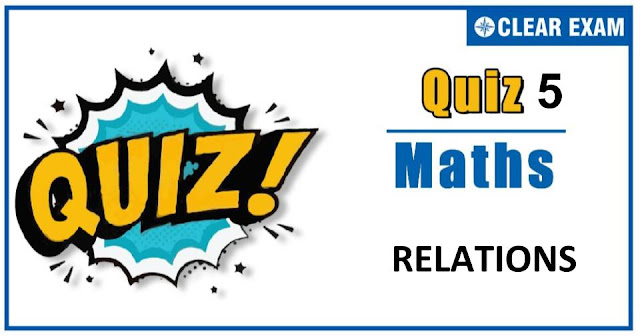## MATHEMATICS RELATIONS QUIZ-5Dear Readers,As per analysis for previous years, it has been observed that students preparing for JEE MAINS find Mathematics out of all the sections to be complex to handle and the majority of them are not able to comprehend the reason behind it. This problem arises especially because these aspirants appearing for the examination are more inclined to have a keen interest in Mathematics due to their ENGINEERING background. Furthermore, sections such as Mathematics are dominantly based on theories, laws, numerical in comparison to a section of Engineering which is more of fact-based, Physics, and includes substantial explanations. By using the table given below, you easily and directly access to the topics and respective links of MCQs. Moreover, to make learning smooth and efficient, all the questions come with their supportive solutions to make utilization of time even more productive. Students will be covered for all their studies as the topics are available from basics to even the most advanced.

Q1. If f:R→R and g:R→R are defined by f(x)=2x+3 and g(x)=x2+7, then the values of x such that g(f(x) )=8 are
•  1,2
•  -1,2
•  -1,-2
•  1,-2
Solution
We have, g(f(x) )=8 ⇒g(2x+3)=8 ⇒(2x+3)2+7=8⇒2x+3=±1⇒x=-1,-2

Q2. Let A be a set containing 10 distinct elements, then the total number of distinct function from A to A is
•  1010
•  101
•  210
•  210-1
Solution
Given a set containing 10 distinct elements and f:A→A
Now, every element of a set A can make image in 10 ways.
∴ Total number of ways in which each element make images =1010.

Q3.  If the functions f and g are defined by f(x)=3x-4,g(x)=3x+2 for x∈R, respectively then
g-1 (f-1 (5) )=
•  1
•  1/2
•  1/3
•  1/4
Solution
Solution

Q5.If f(x)=sin⁡((log⁡x ),) then the value of f(xy)+f(x/y)-2f(x) cos⁡(log⁡y ), is
•  -1
•  0
•  1
•  None of these
Solution
Q6. 36. The relation R={(1,1),(2,2),(3,3)} on the set {1, 2, 3} is
•  Symmetric only
•  Reflexive only
•  An equivalence relation
•  Transitive only
Solution
The relation R={(1,1),(2,2),(3,3)} on the set {1,2,3} is an equivalent relation.

Q8.If f:R→R is defined by f(x)=x-[x]-1/2 for x∈R, where [x] is the greatest integer not exceeding x, then {x∈R:f(x)=1/2} is equal to
•  Z, the set of all integers
•  N, the set of all natural numbers
•  ϕ, the empty set
•  R
Solution

Q9.Consider the following relations R={(x,y)│x, y are real numbers and x=wy for some rational number w};S={(m/n,p/q)|m,n,p and q are integers such that n,q≠0 and qm=pn}. Then
•  R is an equivalence relation but S is not an equivalence relation
•  Neither R nor S is an equivalence relation
•  S is an equivalence relation but R is not an equivalence relation
•  R and S both are equivalence relations
Solution

Q10.
•  [4, 6]
•  (-∞,6)
•  [2, 3)
•  None of these
Solution
For f(x) to be defined, x-4≥0 and 6-x≥0 ⇒ x≥4 and x≤6 Therefore, the domain is [4, 6].#### Written by: AUTHORNAME

AUTHORDESCRIPTION## Want to know more

Please fill in the details below:

## Latest NEET Articles\$type=three\$c=3\$author=hide\$comment=hide\$rm=hide\$date=hide\$snippet=hide

Name

ltr
item
BEST NEET COACHING CENTER | BEST IIT JEE COACHING INSTITUTE | BEST NEET & IIT JEE COACHING: RELATIONS-QUIZ-5
RELATIONS-QUIZ-5
https://1.bp.blogspot.com/-GGy00l4Sio4/X3_QpAvTOrI/AAAAAAAABVA/JorRAPXkX4ow5V7QbpD_gMz_eavHTAw2ACLcBGAsYHQ/w640-h336/Quiz%2BImage%2BTemplate%2B%252812%2529.jpg
https://1.bp.blogspot.com/-GGy00l4Sio4/X3_QpAvTOrI/AAAAAAAABVA/JorRAPXkX4ow5V7QbpD_gMz_eavHTAw2ACLcBGAsYHQ/s72-w640-c-h336/Quiz%2BImage%2BTemplate%2B%252812%2529.jpg
BEST NEET COACHING CENTER | BEST IIT JEE COACHING INSTITUTE | BEST NEET & IIT JEE COACHING
https://www.cleariitmedical.com/2020/10/RELATIONS-QUIZ-5.html
https://www.cleariitmedical.com/
https://www.cleariitmedical.com/
https://www.cleariitmedical.com/2020/10/RELATIONS-QUIZ-5.html
true
7783647550433378923
UTF-8

STAY CONNECTED